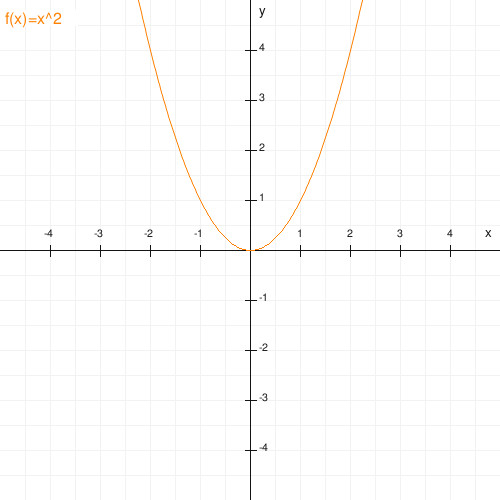## whoami(1)

Physicist, lecturer in maths and physics.

## repositories

plotter is a PHP function plotter able to draw curves of mathematical expressions as images. Here's an example what these plotter images might look like...### mathematics

some packages I used in math education: using a html5 canvas to create plots of simple things dynamically (license is MIT as some additional jquery is used):

• CNum.js (github repository) is a JavaScript class to visualize complex numbers in a z-plane (a gaussian plot). Some lines of JavaScript will create nice gaussian plots on the fly (handles addition and multiplication of complex numbers).
• LNum.js (github repository is a JavaScript class to visualize integers (including natural numbers, of course) and rationals on a number line. This sounds a bit simple simple, but it is quite useful.